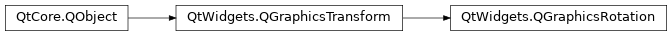# QGraphicsRotation¶

The `QGraphicsRotation` class provides a rotation transformation around a given axis. MoreNew in version 4.6.

## Detailed Description¶

You can provide the desired axis by assigning a `QVector3D` to the axis property or by passing a member if `Axis` to the `setAxis` convenience function. By default the axis is (0, 0, 1) i.e., rotation around the Z axis.

The angle property, which is provided by `QGraphicsRotation` , now describes the number of degrees to rotate around this axis.

`QGraphicsRotation` provides certain parameters to help control how the rotation should be applied.

The origin is the point that the item is rotated around (i.e., it stays fixed relative to the parent as the rest of the item is rotated). By default the origin is `QPointF` (0, 0).

The angle property provides the number of degrees to rotate the item clockwise around the origin. This value also be negative, indicating a counter-clockwise rotation. For animation purposes it may also be useful to provide rotation angles exceeding (-360, 360) degrees, for instance to animate how an item rotates several times.

Note: the final rotation is the combined effect of a rotation in 3D space followed by a projection back to 2D. If several rotations are performed in succession, they will not behave as expected unless they were all around the Z axis.

class PySide2.QtWidgets.QGraphicsRotation([parent=None])

Constructs a new `QGraphicsRotation` with the given `parent` .

PySide2.QtWidgets.QGraphicsRotation.angle()
Return type:

float

This property holds the angle for clockwise rotation, in degrees..

The angle can be any real number; the default value is 0.0. A value of 180 will rotate 180 degrees, clockwise. If you provide a negative number, the item will be rotated counter-clockwise. Normally the rotation angle will be in the range (-360, 360), but you can also provide numbers outside of this range (e.g., a angle of 370 degrees gives the same result as 10 degrees). Setting the angle to NaN results in no rotation.

origin

PySide2.QtWidgets.QGraphicsRotation.angleChanged()
PySide2.QtWidgets.QGraphicsRotation.axis()
Return type:

`PySide2.QtGui.QVector3D`

This property holds a rotation axis, specified by a vector in 3D space..

This can be any axis in 3D space. By default the axis is (0, 0, 1), which is aligned with the Z axis. If you provide another axis, `QGraphicsRotation` will provide a transformation that rotates around this axis. For example, if you would like to rotate an item around its X axis, you could pass (1, 0, 0) as the axis.

`QTransform` `angle`

PySide2.QtWidgets.QGraphicsRotation.axisChanged()
PySide2.QtWidgets.QGraphicsRotation.origin()
Return type:

`PySide2.QtGui.QVector3D`

This property holds the origin of the rotation in 3D space..

All rotations will be done relative to this point (i.e., this point will stay fixed, relative to the parent, when the item is rotated).

PySide2.QtWidgets.QGraphicsRotation.originChanged()
PySide2.QtWidgets.QGraphicsRotation.setAngle(arg__1)
Parameters:

arg__1 – float

This property holds the angle for clockwise rotation, in degrees..

The angle can be any real number; the default value is 0.0. A value of 180 will rotate 180 degrees, clockwise. If you provide a negative number, the item will be rotated counter-clockwise. Normally the rotation angle will be in the range (-360, 360), but you can also provide numbers outside of this range (e.g., a angle of 370 degrees gives the same result as 10 degrees). Setting the angle to NaN results in no rotation.

origin

PySide2.QtWidgets.QGraphicsRotation.setAxis(axis)
Parameters:

axis`Axis`

Convenience function to set the axis to `axis` .

Note: the `YAxis` rotation for `QTransform` is inverted from the correct mathematical rotation in 3D space. The `QGraphicsRotation` class implements a correct mathematical rotation. The following two sequences of code will perform the same transformation:

```QTransform t;
t.rotate(45, Qt::YAxis);

QGraphicsRotation r;
r.setAxis(Qt::YAxis);
r.setAngle(-45);
```
PySide2.QtWidgets.QGraphicsRotation.setAxis(axis)
Parameters:

This property holds a rotation axis, specified by a vector in 3D space..

This can be any axis in 3D space. By default the axis is (0, 0, 1), which is aligned with the Z axis. If you provide another axis, `QGraphicsRotation` will provide a transformation that rotates around this axis. For example, if you would like to rotate an item around its X axis, you could pass (1, 0, 0) as the axis.

`QTransform` `angle`